Thread: Eric Dollard View Single Postt-rexSenior Member Join Date: Aug 2011 Location: In the bushes Posts: 422
The Law of Electro-Magnetic Induction, Part 5. (1 of 2)

(1) This part in the series, Law of Electro-Magnetic Induction here continues with chapter three from the Steinmetz A.C.. book, 1916 edition. Here he has just given his definition of the Volt as,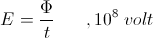And by algebraic operation, the magnetic charge is,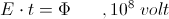The Time Interval, t, is one second, and the quantity of induction,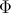, is one hundred mega-lines of flux. This relation defines one volt of electro-motive force. In the next paragraph, page 16, Steinmetz turns the eighth power around, this to the negative eight, altering the relation to,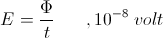Compounded on this relation is the number of turns in the coiled winding,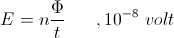And hereby it is,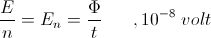Where En is the volt per turn and n is the number of turns. No explanation is provided as to the reason for the reversal of the power of ten, from plus eight, to minus eight. We are off to a good start here!

The number of turns in the winding act co-jointly to multiply the E.M.F. These turns also act co-jointly to the multiply the current. This current, i, in amperes, is continuous throughout the coiled winding. As each turn comes about it carries current, i, with it, round and round again, throughout the total number of turns. The net result is a sheet current, this consisting of n individual currents. This is given by the relation,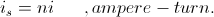This sheet current is the magneto-motive force that maintains the magnetic induction. This is in distinction to the current, i, which is related to the electronic current. Hence the M.M.F. is expressed in ampere-turns, the electronic current, i, and the number of turns, n. For example, a reactance coil has 1000 turns, and it is drawing a current, i, of one ampere, the sheet current of the coil, is, is now 1000 amperes. Hence this coil is operating with a M.M.F. of 1000 ampere-turns. Large M.M.F.'s can be produced with small currents thru the compounding of these currents via multiple turn windings of many turns. The limiting factor is the accumulation of series resistance as the winding gets longer in length.

This was an important discovery in electro-magnetism. It's first engineering application was the Morse Telegraph Coil as developed by Joseph Henry, the American Faraday.

(2) These multiple turns not only multiply the current, i, they also multiply the E.M.F., E. The currents compound, side by side, in a parallel fashion, this creating a sheet current. The E.M.F. per turn compounds, end to end, in a series fashion. Each and every turn develops an identical E.M.F. All turns are linked together thru the mutual magnetic induction which surrounds the entire winding. Each individual E.M.F. adds to the next, in a series manner, developing a total E.M.F., that of the entire winding.

This is expressed in the relation,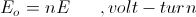Where,

Eo, the total end to end E.M.F.

E, the individual turn E.M.F.

For example, the same reactance coil, 1000 turns. This coil is discharged at a constant rate over a period of one second. During this discharge each turn develops an E.M.F. of one volt. The coil has 1000 turns, hence the total E.M.F. at the ends of the windings is 1000 volts. This is the principle of automobile ignition coil, a magnetic discharge device.

Here established is two relations, one for the total M.M.F.,The other for the total E.M.F.,The individual currents are identical thruout the coiled winding, no gradient exists in this current. The individual E.M.F's are also identical, but not as with the current. A gradient exists in between the turns as the E.M.F. compounds along the winding. Hence a voltage gradient exists along the winding length expressed as volts per turn. Here in this metallic-dielectric geometry the magnetic force and the dielectric force are oriented in the same direction, (MK).
__________________
SUPPORT ERIC DOLLARD'S WORK AT EPD LABORATORIES, INC.

Purchase Eric Dollard's Books & Videos - 70% of the sale goes to Eric and EPD Laboratories: Eric Dollard Books & Videos
Donate by Paypal: Donate to EPD Laboratories

Last edited by t-rex; 07-23-2012 at 11:14 PM.